# Current Divider Circuit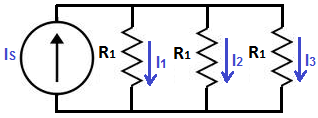In this article, we will go over what current division is, its importance in a circuit, its mathematical formula to calculate how current gets divided in a circuit, and how a current divider fits in circuit design to produce useful electronic roducts. You will learn that a current divider is a very useful concept in order to build real-world products. Many devices operate on this principle. We will even go over, in this article, many electronic products that are designed either partly or wholly based on the laws of current division.

### What is Current Divider Circuit?

A current divider circuit is a circuit in which the main current from the power source is divided up in the circuit and, thus, different amounts of current are allocated to different parts of the circuit.
Look at the below circuit schematic to see current division in action.

### Current Divider with Current Source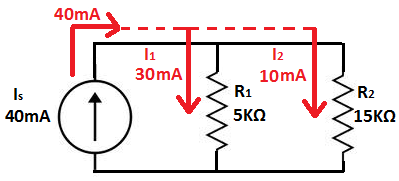The first type of current divider circuit we will go over is one in which the power source is a current source.
You can see how current divides up when a circuit is in parallel, as the two resistors are.
When a circuit has resistors in parallel, the current from the power source (as you can see in this circuit is the 40mA current source) divides up in the parallel resistors.
Current takes the path of least resistance. So most of the current goes through the smaller-valued resistor, which in this case is the 5KΩ resistor. So if you want more current to flow through a particular part of a circuit, put less resistance in that part of the circuit. Therefore, more current will travel throught that part in the circuit. Conversely, if you want less current to flow through another part of a circuit, put more resistance in that part of the circuit. This is how current division works.
Note that current only divides up in a parallel circuits. In series, current is the same across all components.

### Mathematical Formula

Now that you know conceptually how current division works in a circuit, let's go over exactly mathematically how current divides in a circuit based on the current power source of the circuit and the resistance values of the resistors or loads in the circuit.
Current divides up in a circuit based on the formula: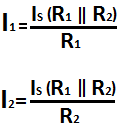The current that goes through a branch in a parallel circuit is equal to the product of the other branch's resistance and the main current source divided by the total resistance of all the branches.
In the above example, the main power source is 40mA.
The current which goes through the branch having the 5KΩ resistor is:

I1= (40mA)(15KΩ)/(5KΩ+15KΩ)= 30mA
The current which goes through the branch having the 15KΩ resistor is:

I2= (40mA)(5KΩ)/(5KΩ+15KΩ)= 10mA

### Current Divider FormulaIf there are more than two branches in parallel, then current can be found according to the current divider formula: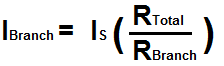where IX is the current going through a certain branch where you are solving for, IS is the current power source, RT is the total equivalent resistance value of the parallel resistor circuit, and RX is the value of the resistance of the branch for the current for which you are solving.

### Current Divider with a Voltage Source

You can also have a current divider circuit with a voltage source as the power source.
This also functions as a current divider, because the voltage source produces current which gets divided up in the circuit when resistors or loads in the circuit are in parallel.
Below is a current divider circuit with a voltage source: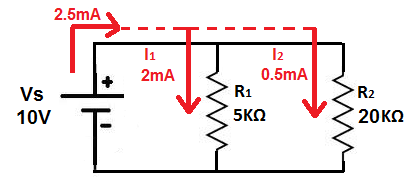Just like before, current takes the path of least resistance, so most of the current goes through the 5KΩ instead of the 20KΩ resistor.

### Mathematical Formula

To find the value of the currents going through the individual parallel branches of a circuit, it is even simpler than a current source.
All we need to do to calculate current values with a voltage source is to use the current formula according to ohm's law: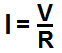Current equals voltage divided by resistance.
In the above example, the main voltage power source is 10V.
The current which goes through the branch having the 5KΩ resistor is then:

I1= 10V/5KΩ= 2mA
The current which goes through the branch having the 20KΩ resistor is:

I2= 10V/20KΩ= 0.5mA
A check you can do to find the total current in the circuit with a voltage source is to find the equivalent resistance value of all the resistors in parallel and then plug that value into the above formula. This will give the total current in the circuit before the current is divided into the individual parallel branches.
If you want to calculate current division in a circuit, visit our Current Divider Calculator . This calculator allows you to calculate the current which goes through any branch in a parallel circuit.